Author: Oscar Cronquist Article last updated on February 08, 2019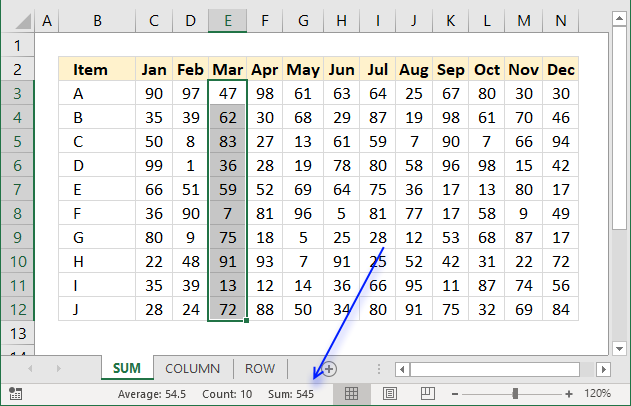The easiest way to sum a cell range is to simply select the cell range and read the values in the status bar. It shows the total, the count of non-empty cells and the average.

In fact, you can customize the status bar to show even more data:

Here is how to show these calculations automatically in the status bar.

1. Press with right mouse button on on the status bar with your mouse.
2. Press with left mouse button on "Numerical Count", "Minimum", and "Maximum", see image below.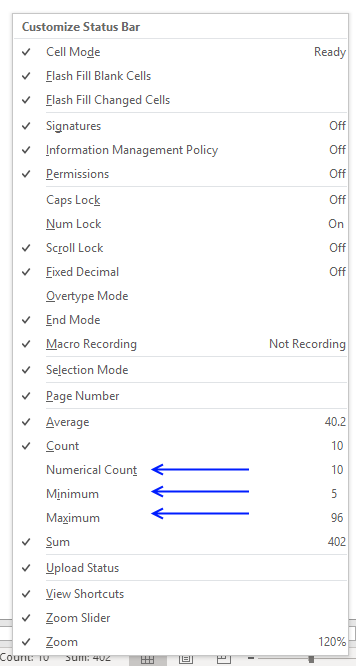The image below demonstrates these calculations enabled.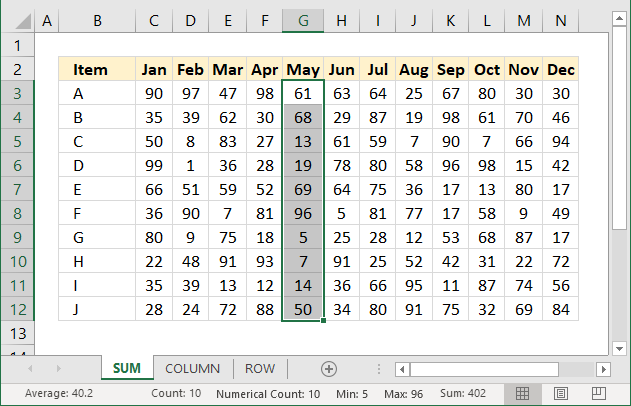### Sum a column using a formula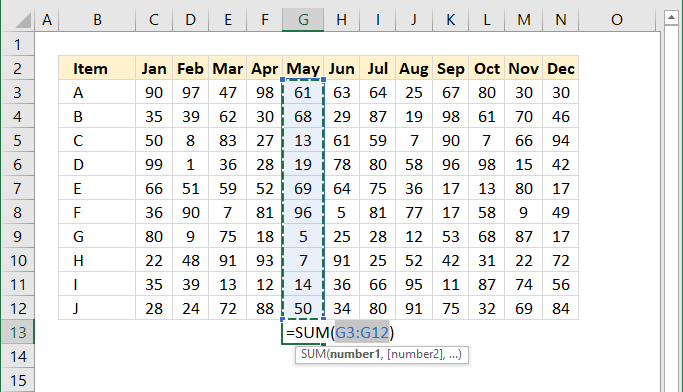This is probably the most common task in Excel and luckily, there is an easy short cut to use.

1. Select the cell range you want to sum.
2. Press and hold Alt on your keyboard.
3. Then press =

This will create a formula containing the SUM function and a cell reference to the selected cells, see image above.

You can also go to tab "Home" on the ribbon and press with left mouse button on "AutoSum" button and get the same result. To create totals below all columns select cell range C13:N13 and press and hold Alt and then press =

### Sum a given column using a formula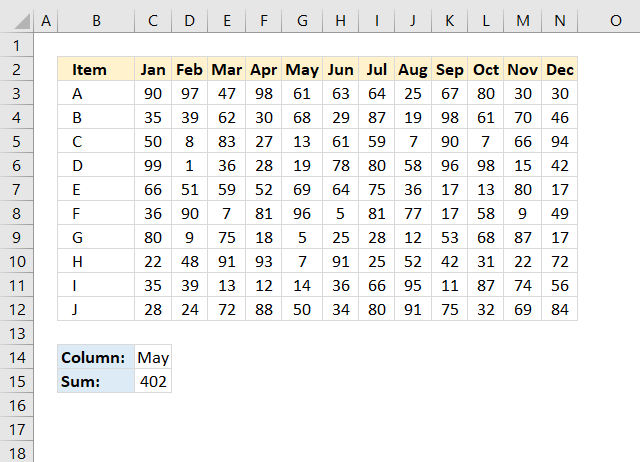The picture above shows a formula in cell C15 that sums a column in cell range C3:N12 based on the specified column header in cell C14.

Formula in cell C15:

=SUM(INDEX(C3:N12, 0, MATCH(C14, C2:N2, 0)))

#### Explaining formula in cell C15

The MATCH function returns a number representing the position of the given value in cell C14, in C2:N2.

MATCH(C14, C2:N2, 0)

becomes

MATCH("May", {"Jan", "Feb", "Mar", "Apr", "May", "Jun", "Jul", "Aug", "Sep", "Oct", "Nov", "Dec"}, 0)

and returns 5.

The INDEX function returns the entire column in cell C3:N12 if the row argument is 0 (zero).

INDEX(C3:N12, 0, MATCH(C14, C2:N2, 0))

becomes

INDEX(C3:N12, 0, 5)

and returns {61; 68; 13; 19; 69; 96; 5; 7; 14; 50}.

The SUM function adds the numbers given and returns a total.

SUM(INDEX(C3:N12, 0, MATCH(C14, C2:N2, 0)))

becomes

SUM({61; 68; 13; 19; 69; 96; 5; 7; 14; 50})

and returns 402.

### Sum a given row using a formula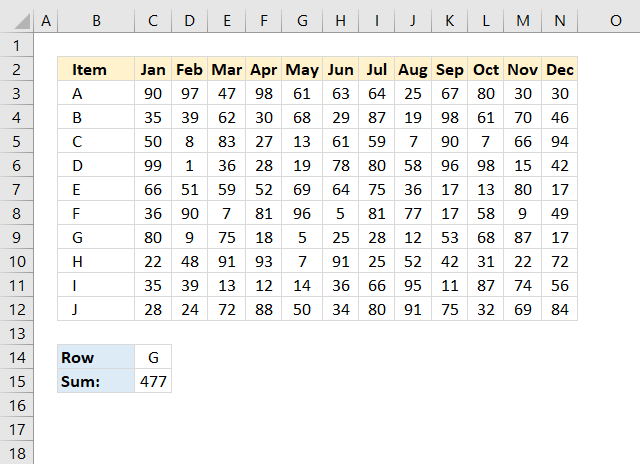Formula in cell C15:

=SUM(INDEX(\$C\$3:\$N\$12,MATCH(C14,B3:B12,0),0))

This formula is very similar to the prior one, no explanation is needed.

### Sum using an Excel defined Table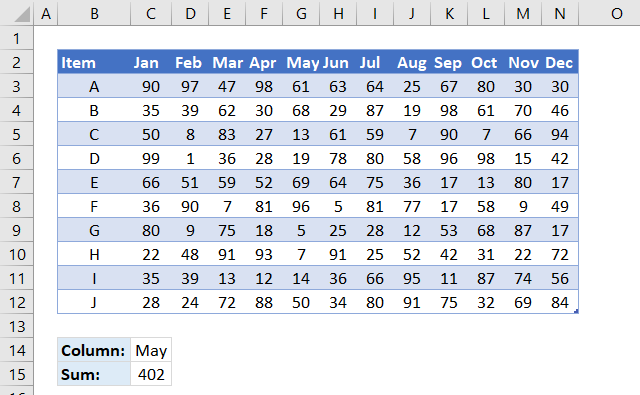This image displays the dataset converted to an Excel defined Table. When you press with left mouse button on and drag to create cell references they are instantly changed to structured references, this means that you generally don't have to adjust the cell references when you add data to the Excel defined Table.

Formula in cell C15: